# How to Find the Area and PerimeterIf you need help with perimeters of a rectangle, go here.

Hey guys! Welcome to this video on finding the area and perimeter of an object.

The area and perimeter help us to measure two-dimensional shapes.

The area measures the surface of an object, and the perimeter measures the length of the outside of an object.

For instance, let’s say you have a rectangular pool.You want to find a tarp to cover it, then you would need to know the area of the surface of the pool (everything blue). But, if you wanted to know the distance it would take for you to walk around the pool, then you would need to know the perimeter.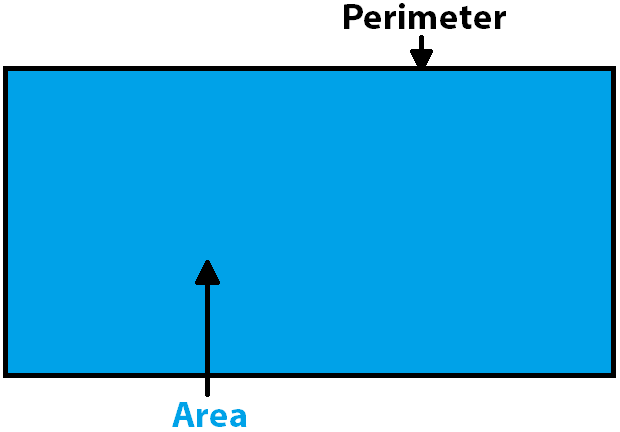Now, let’s take a look at how to calculate each one.

## How to find Perimeter

First, perimeter. To calculate the perimeter of any polygon you just add up the length of all the sides.

Let’s use our rectangular pool as an example. Let’s say that the width is 15 feet, and the length is 7 feet.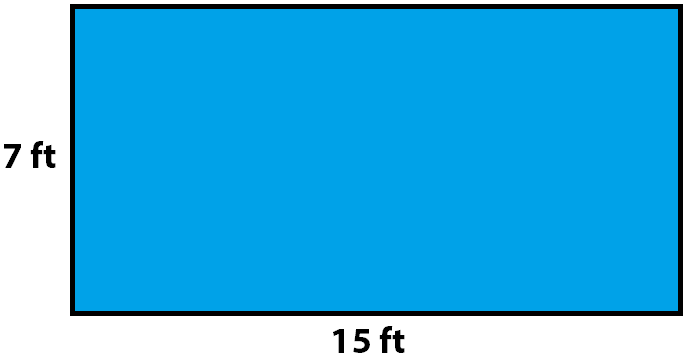Since it is a rectangle we know that the parallel sides have the same length.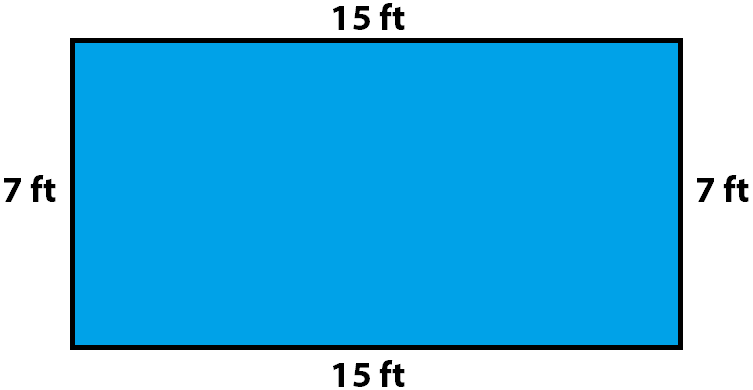So, we have all the information we need. Now just add up all the sides.

Let’s start with our formula. Let’s say 15 is equal to $$a$$, and 7 is equal to $$b$$.

So we have $$P= a + a + b + b$$.

Now let’s plug in our numbers. $$P=15\text{ ft}+15\text{ ft}+7\text{ ft}+7\text{ ft}$$. So we add it all up we get our perimeter is equal to 44 feet.

We can even simplify our perimeter formula for a rectangle. Since we know that there are 4 sides and that there are two sets of identical sides (the two sides that are parallel to each other), we can then simplify our formula to $$P=2(a+b)$$. Let’s try and see if we get the same thing.

$$P=2(15\text{ ft}+7\text{ ft})=2(22\text{ ft})=44\text{ ft}$$

So, you can see here that we do, indeed, get the same thing.

We can apply this same sort of principle to finding the perimeter of a square. Since, a square has four sides that are the same measurement, we can say that the perimeter of a square is $$4\times a$$.

The same principle is true with an equilateral triangle. All three sides are the same so we can say $$P=3\times a$$. An Isosceles triangle has two sides that are the same so we can write our perimeter formula as $$P=2a+b$$.

Now, the only shape whose perimeter may seem a little less obvious to find is our friend the circle. You guys may have heard of the term circumference. Well, the circumference is the exact same thing as the perimeter of a circle.

Some mathematicians may get a little on edge about which term you use, because technically, perimeter is defined as “the sum of the lengths of the edges of a closed figure,” and a circle doesn’t have edges. But the definition for circumference is the perimeter of a circle. So, whichever way you prefer to call it, let’s look at how you find it.

To find the circumference of a circle you multiply the diameter times $$π$$: ($$C=d\pi$$).

Alright, now on to area.

## How to find Area

When we were looking at the perimeter of different shapes we were able to simplify the formulas. Giving us different formulas for different shapes, yet keeping in line with the definition of adding up all the sides.

Well, similarly, when finding the area of an object the formula will be different for different shapes, but each formula represents a solution to solving for the area of the object’s surface.

So, let’s take a look at the different shapes, and the area formula corresponding for each shape.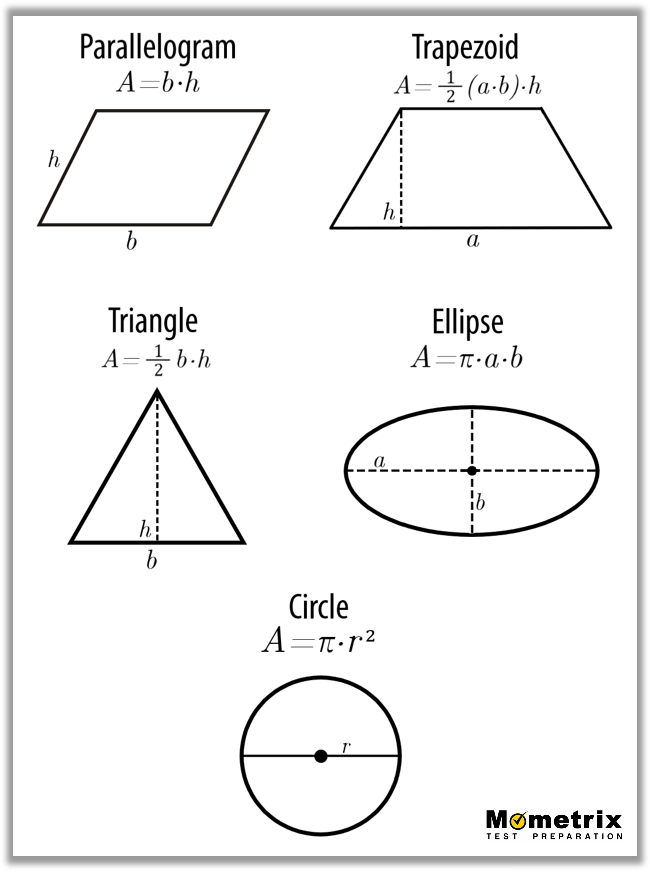Okay, now let’s look at some examples of how to solve for the area of an ellipse, and the area of a trapezoid.

Let’s say you have an ellipse and $$a=6$$, and $$b=4$$. Now, let’s plug in our numbers into the formula for an ellipse.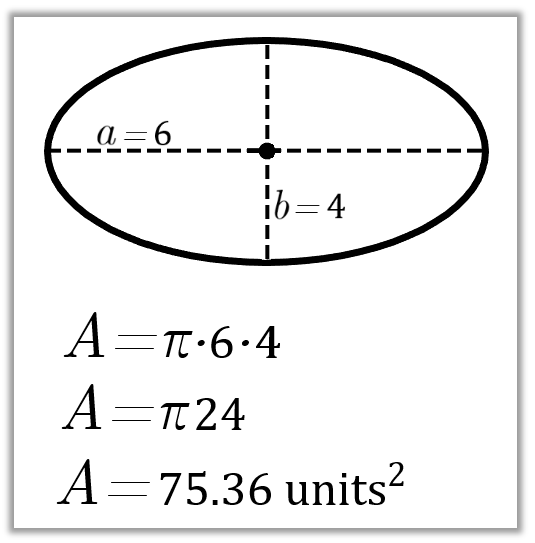Now, let’s say we have a trapezoid. We’ll say that our height is equal to 7, our $$a$$ side is equal to 8, and our $$b$$ side is equal to 5. Now, we do the exact same thing that we did last time. We just plug in our numbers into our formula for area.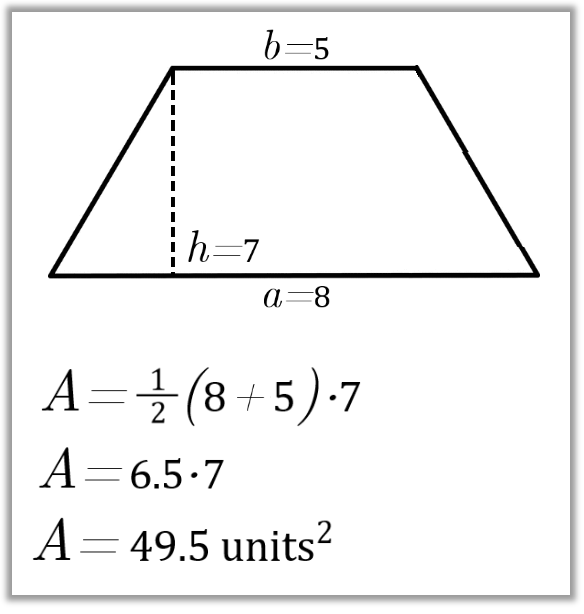Alright guys, I hope that this video has been helpful.

See you guys next time!

### How do you find area?

#### A

Each shape has a unique area formula. Here are some of the most common:
Circle: $$A=πr^2$$
Square: $$A=s^2$$
Rectangle: $$A=lw$$
Triangle: $$A=\frac{1}{2}bh$$
Parallelogram: $$A=bh$$
Trapezoid: $$A=1/2 (b_1+b_2)h$$

### How do you find the perimeter?

#### A

Find perimeter by adding together the lengths of each side of the figure.
Ex. What is the perimeter of this figure?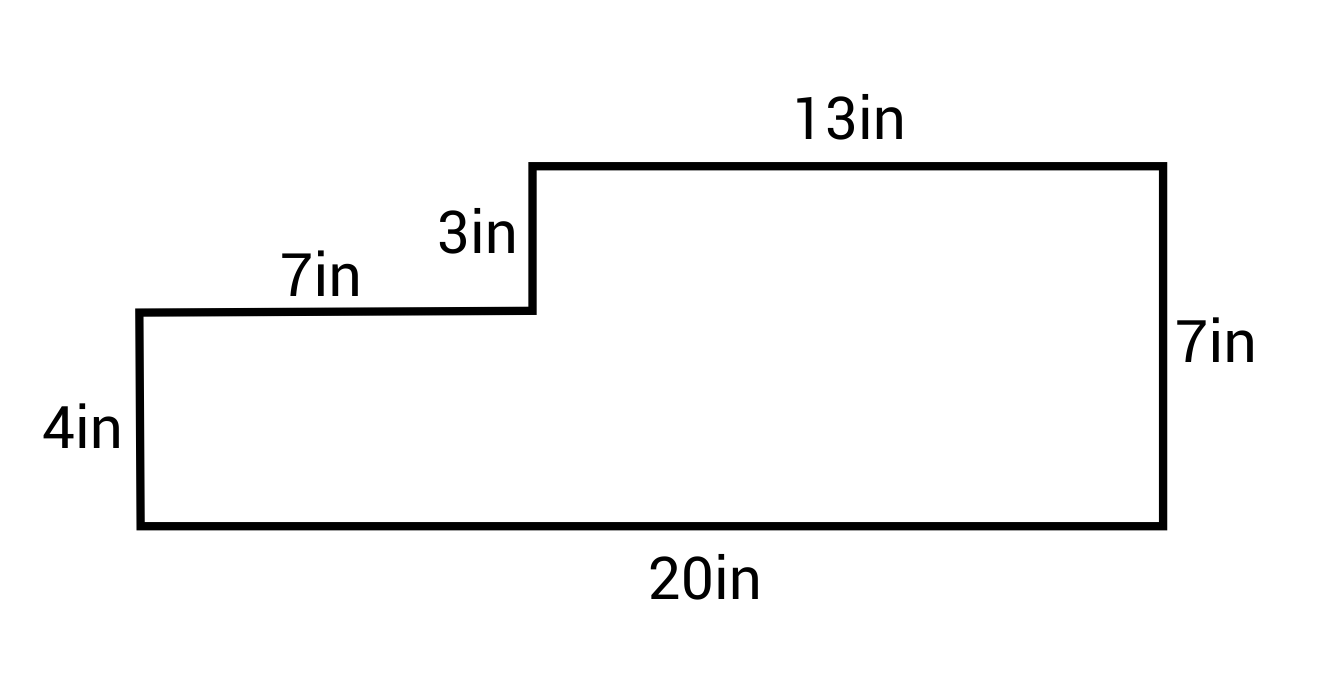P = 4 + 7 + 3 + 13 + 7 + 20 = 54 in

### How do you find the area of a triangle?

#### A

The most common way to find the area of a triangle is by multiplying its base times its height and dividing by 2.
$$A=\frac{1}{2}bh$$
Some other triangle area formulas are:
Any triangle: $$A=\sqrt{s(s-a)(s-b)(s-c)}$$, where s is the semi-perimeter (half the perimeter), and a, b, and c are side lengths.
Isosceles triangle: $$A=\frac{1}{2}b \sqrt{(a^2-\frac{b^2}{4})}$$, where b is the unique side and a is the length of one of the two congruent sides.
Equilateral triangle: $$A=\frac{\sqrt{3}}{4}a^2$$, where a is the length of a side.
Ex. What is the area of this triangle?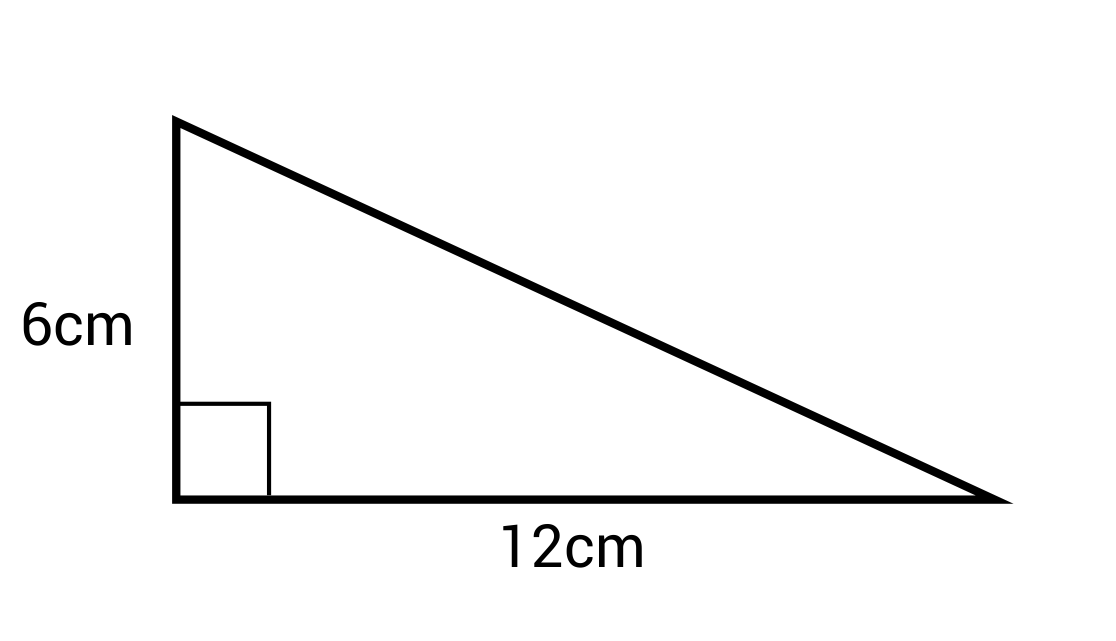$$A=\frac{1}{2} bh=\frac{1}{2} (12)(6)=36 cm^2$$

### How do you find the perimeter of a rectangle?

#### A

Find the perimeter of a rectangle by multiplying its length by 2 and its width by 2 and adding the values, or by adding its length and width and then multiplying by 2.
P = 2l + 2w or P = 2(l + w)
Ex. What is the perimeter of this rectangle?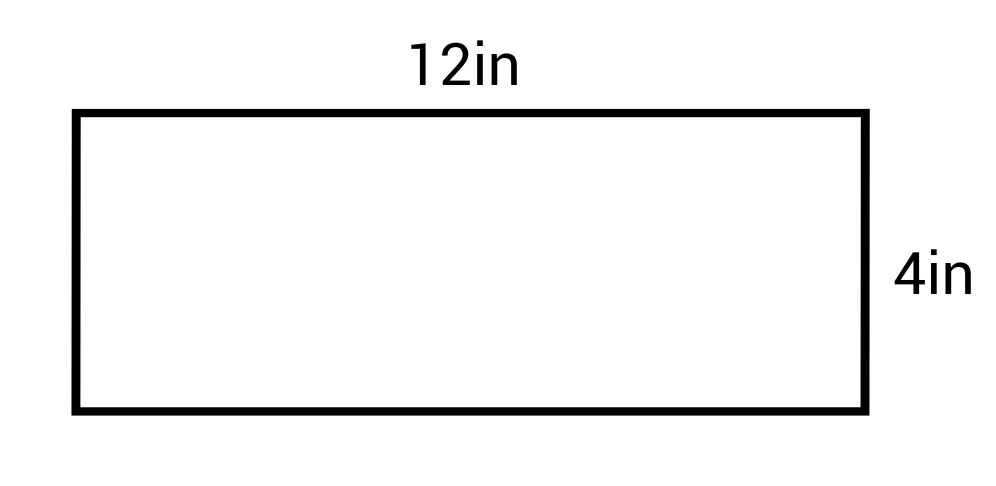P = 2(12) + 2(4) = 24 + 8 = 32 in or P = 2(12 + 4) = 2(16) = 32 in

### How do you find the area of a circle?

#### A

Find the area of a circle by multiplying pi (π) times the radius squared.
$$A=πr^2$$
Ex. What is the area of this circle?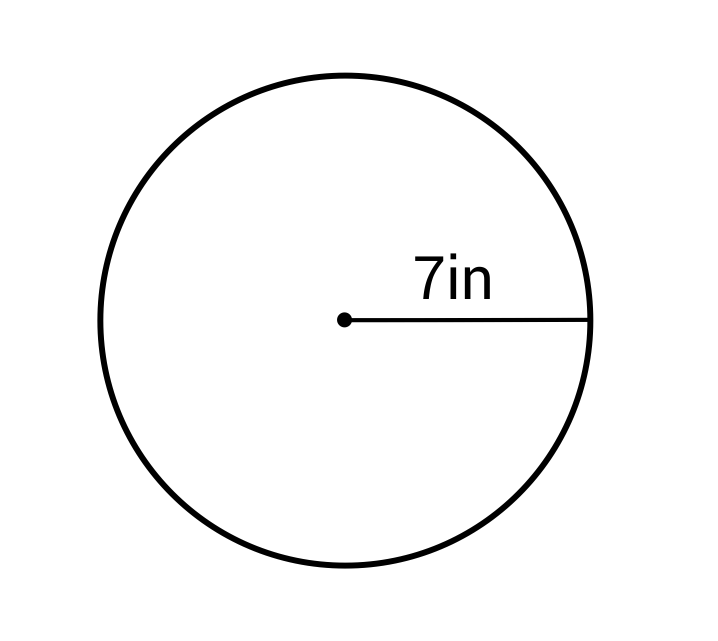$$A=π(7)^2=49πin^2≈156.86in^2$$

### How do you find the perimeter of a triangle?

#### A

Find the perimeter of a triangle by adding the lengths of all three sides together.

## Practice Questions

Question #1:

What is the area of this rectangle?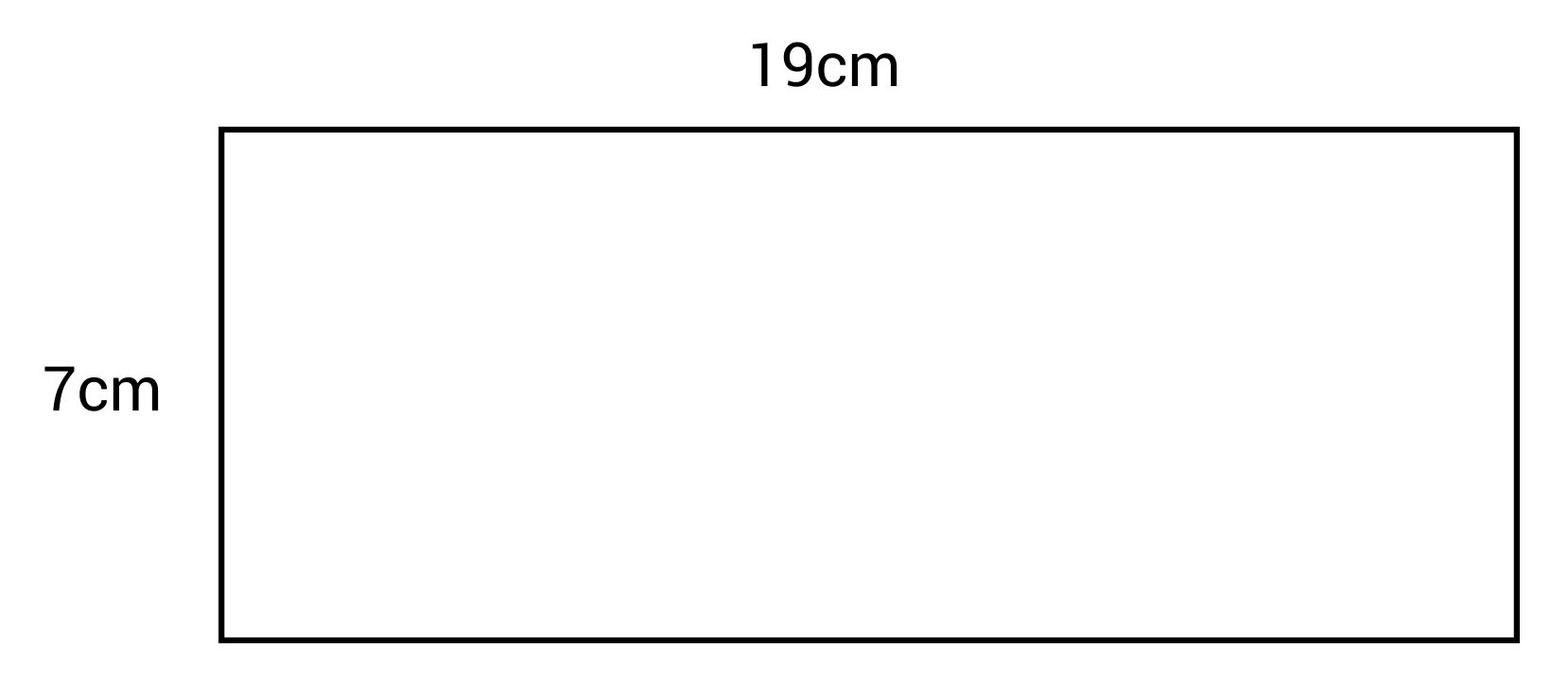52 cm2

133 cm2

47 cm2

154 cm2

The correct answer is 133 cm. To find the area of a rectangle, multiply its length by its width.
$$A=lw$$
The length is 19 cm and the width is 7 cm.
$$A=19\times7=133\text{ cm}^2$$

Question #2:

What is the area of a square with a side length of 4 in?

28 in2

24 in2

12 in2

16 in2

The correct answer is 16 in2. To find the area of a square, square the length of its side.
$$A=s^2$$
$$A=(4)^2=16\text{ in}^2$$

Question #3:

What is the perimeter of this rectangle?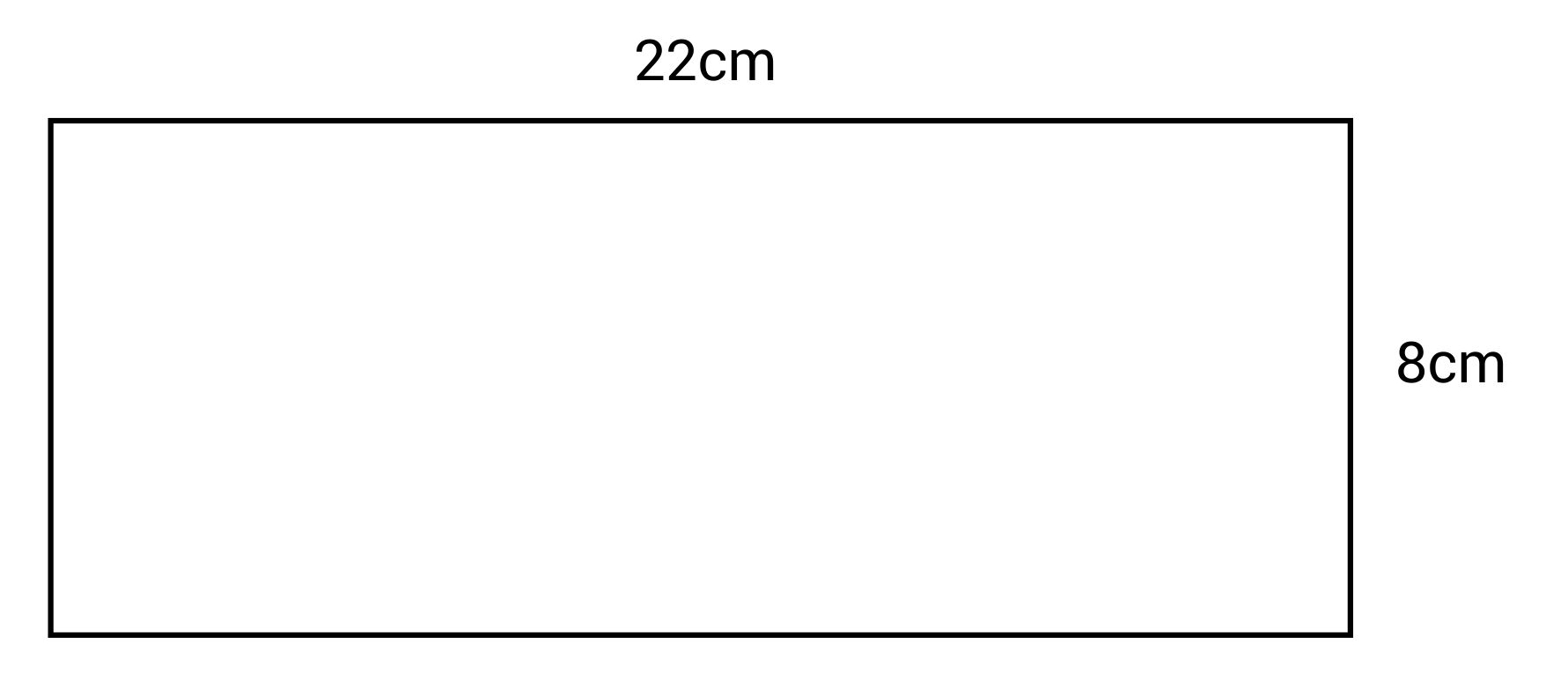192 cm

176 cm

60 cm

30 cm

The correct answer is 60 cm. The formula for perimeter of a rectangle is:
$$P=2(l+w)$$ or
$$P=2l+2w$$
The length is 22 cm and the width is 8cm.
$$P=2(22+8)=2(30)=60\text{ cm}$$

Question #4:

What is the perimeter of this pentagon?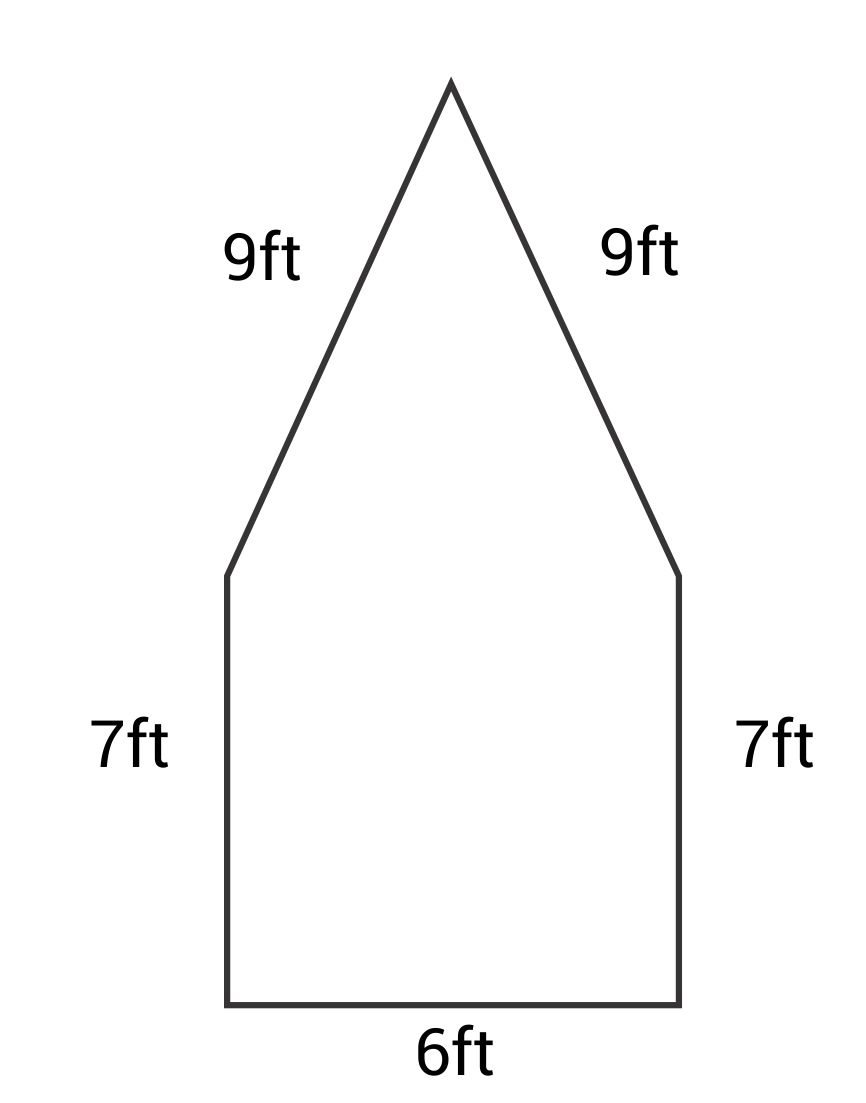38 ft

26 ft

42 ft

59 ft

The correct answer is 38 ft. Find the perimeter of a figure by adding the lengths of all the sides together.
$$P=9+9+7+6+7=38\text{ ft}$$

Question #5:

What is the formula for finding the perimeter of a rectangle?

$$P=4s$$
$$P=2a+b+c$$
$$P=a+b+c$$
$$P=2l+2w$$
The correct answer is $$P=2l+2w$$. Find the perimeter of a rectangle by adding double the length plus double the width, or add the length and width together and then double that number.